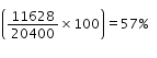# Three candidates contested in an election and received 1136, 7636

Three candidates contested in an election and received 1136, 7636 and 11628 votes respectively. What percentage of the total votes did the winning candidate get?

Total number of votes polled = (1136 + 7636 + 11628) = 20400.
Maximum votes of winning candidate = 11628
Therefore, required percentage =